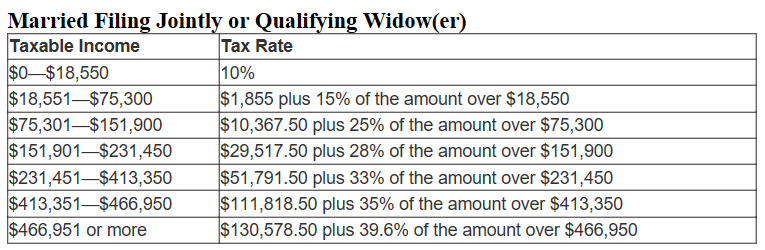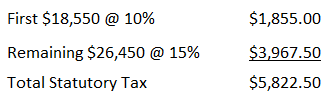# Effective Tax Rate

## Definition of Effective Tax Rate:

The effective tax rate is the average tax rate paid after considering all tax deductions and tax credits.

## Detailed Explanation of Effective Tax Rate:

The formula used to calculate the effective tax rate for individuals equals the total tax paid divided by total income. This quotient is then multiplied by 100 to convert it to a percentage. The total income for US tax payers can be found on Line 22 of the IRS Form 1040. Note that deductions have not been subtracted at this point on the tax return.

Effective Tax Rate = (Total Tax Paid / Total Income) x 100

(For corporations the formula differs slightly from individuals in that the profit before tax is the denominator. This means that operating expenses have been already been deducted.)

It is important to distinguish between the statutory rate, effective rate, and marginal rate. Statutory rates are set by the statutes and do not consider tax benefits from tax credits that are subtracted directly from taxable income. Let's use an example to illustrate the differences. Assume you are married and recently graduated from college. You and your spouse both work and have a combined income of \$55,000 before tax. You have \$10,000 in deductions, resulting in a taxable income of \$45,000. Taxable income is gross income less deductions. (In the United States, it is found on Line 43 of Tax Form 1040.) The tax table providing the statutory tax rates for 2016 married couples filing jointly is below.The statutory rate is 10 percent for the first \$18,850 you earn. You owe \$1,855 on this portion of your income. For the remaining taxable income (\$26,450) the statutory rate equals 15 percent, so you would owe \$3,967.50 on the taxable income exceeding \$18,550. Your total statutory income tax equals \$5,822.50.Most governments have tax credits and tax deductions that reduce a taxpayer’s tax. They are enacted to either provide assistance to a group of people or an incentive for a particular behavior. For example, in the United States, the Credit for Child and Dependent Care Services (Line 49 of 1040) is a tax credit to provide an incentive for parents to work and lower their after-tax expense for day-care. A tax credit is subtracted from the statutory tax derived from the tax tables.

The effective rate is an average rate – and is calculated by taking the tax paid and dividing it by the income. Continuing with our example, assume you have one child. Last year you paid for child care and the government provides you with a \$1,000 credit to help you. Your tax liability is reduced to \$4,822.50. Your effective tax rate equals your tax liability divided by your total income (Line 22 on Form 1040), or 8.77 percent. (For corporations, the formula would be their tax liability divided by the profit before tax.)

(\$4,822.50 / \$55,000) *100 = 8.77%

The effective tax is a historical tax rate because it is determined by past activities. The marginal tax rate is the tax rate that would be paid on the next dollar earned, so it is forward-looking. For this reason, economists favor the marginal tax rate when making financial decisions. For individuals, it is normally the taxpayer’s tax bracket. Continuing with our example, assume you received a \$100 bonus. You would remain in the 15 percent tax bracket so you pay an additional \$15 in tax. Your marginal tax rate equals 15 percent. This is the most appropriate rate when determining investment decisions such as investing in a tax-free municipal bond.